# FTCE Geometric Concepts Flashcards

FTCE Geometric Concepts Flashcards
1/112 (missed) 0 0
Create Your Account To Continue Studying

As a member, you'll also get unlimited access to over 79,000 lessons in math, English, science, history, and more. Plus, get practice tests, quizzes, and personalized coaching to help you succeed.

Try it risk-free for 30 days. Cancel anytime
Describe how you would find the area of a composite figure.
Divide the shape into smaller shapes where the area of each can be found easily. Add up the area of each smaller figure to find the area of the entire figure.
Got it
Fill in the blank with the appropriate word: When measuring area, the units will always be given in ________ units.
Square
Got it
Give the area of an ellipse formula in terms of a and b, where a represents the radius of the major axis and b represents the radius of the minor axis.
The formula is: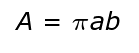Got it
Give the area of a trapezoid formula in terms of a, b, and h, where a and b represent the lengths of the two bases and h represents the height.
The formula is: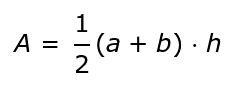Got it
Give the area of a parallelogram formula in terms of b and h, where b represents the length of the base and h represents the height.
The formula is: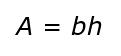Got it
Give the area of a circle formula in terms of r, where r represents the radius.
The formula is: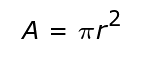Got it
Give the area of a triangle formula given in terms of b and h, where b is the base and h is the height.
The formula is: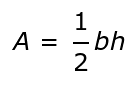Got it
Give the area of a square formula in terms of s, where s stands for side length.
The formula is: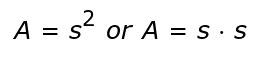Got it
Give the area of a rectangle formula in terms of l and w, where l stands for length and w stands for width.
The formula is: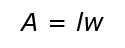Got it
Area
The amount of space taken up by a two-dimensional object.
Got it
Cheryl wants to outline her pentagon-shaped garden with daises. If each side is 2 feet long, find the perimeter of the garden.
The perimeter is 10 feet.
Got it
Find the perimeter of the following: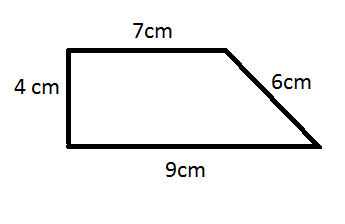The perimeter is 26 cm.
Got it
Find the side length of a square with a perimeter of 28 units.
The side length of this square would be 7 units.
Got it
Composite Figure
A figure made of two or more shapes.
Got it
Give the formula for the perimeter of a triangle in terms of s, where s stands for each side length.
The formula is: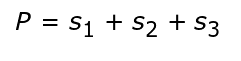Got it
Give the formula for the perimeter of a rectangle in terms of l and w, given that l stands for length and w stands for width.
The formula is: P = 2(l+w) or P = 2l + 2w
Got it
Give the formula for the perimeter of a square in terms of s, where s stands for side length.
The formula is: P = 4s
Got it
Circumference

The perimeter of a circle. The formula is C = 2?r, where r is the radius.

Got it
Polygon
A two-dimensional closed-in figure with straight sides and angles.
Got it
Perimeter
The total length around an area or shape.
Got it

or choose a specific lesson: See all lessons in this chapter
112 cards in set

## Flashcard Content Overview

The study of shapes is one of the main focuses of geometry for students in grades K-6. They begin by learning how to identify basic shapes. This set will test you on not only picture identification of the two- and three-dimensional shapes but on their definitions as well. After mastering identification, K-6 students then learn how to measure different components of each shape. When given a two-dimensional shape, you must be able to calculate perimeter and area. When given a three-dimensional shape, you must be able to calculate surface area and volume. Euler's formula can even be applied to the simple act of counting faces, edges, and vertices for the older or more advanced learner. This flashcard leaves no stone unturned when it comes to the study of shapes and promises to give you practice on every topic you will need to master for geometric concept portion of the the Florida Teacher Certification Exam.

Front
Back
Perimeter
The total length around an area or shape.
Polygon
A two-dimensional closed-in figure with straight sides and angles.
Circumference

The perimeter of a circle. The formula is C = 2?r, where r is the radius.

Give the formula for the perimeter of a square in terms of s, where s stands for side length.
The formula is: P = 4s
Give the formula for the perimeter of a rectangle in terms of l and w, given that l stands for length and w stands for width.
The formula is: P = 2(l+w) or P = 2l + 2w
Give the formula for the perimeter of a triangle in terms of s, where s stands for each side length.
The formula is:Composite Figure
A figure made of two or more shapes.
Find the side length of a square with a perimeter of 28 units.
The side length of this square would be 7 units.
Find the perimeter of the following:The perimeter is 26 cm.
Cheryl wants to outline her pentagon-shaped garden with daises. If each side is 2 feet long, find the perimeter of the garden.
The perimeter is 10 feet.
Area
The amount of space taken up by a two-dimensional object.
Give the area of a rectangle formula in terms of l and w, where l stands for length and w stands for width.
The formula is:Give the area of a square formula in terms of s, where s stands for side length.
The formula is:Give the area of a triangle formula given in terms of b and h, where b is the base and h is the height.
The formula is:Give the area of a circle formula in terms of r, where r represents the radius.
The formula is:Give the area of a parallelogram formula in terms of b and h, where b represents the length of the base and h represents the height.
The formula is:Give the area of a trapezoid formula in terms of a, b, and h, where a and b represent the lengths of the two bases and h represents the height.
The formula is:Give the area of an ellipse formula in terms of a and b, where a represents the radius of the major axis and b represents the radius of the minor axis.
The formula is:Fill in the blank with the appropriate word: When measuring area, the units will always be given in ________ units.
Square
Describe how you would find the area of a composite figure.
Divide the shape into smaller shapes where the area of each can be found easily. Add up the area of each smaller figure to find the area of the entire figure.
Find the area of a circle with a radius of 5mm.
78.5 mm2
Find the area of the figure: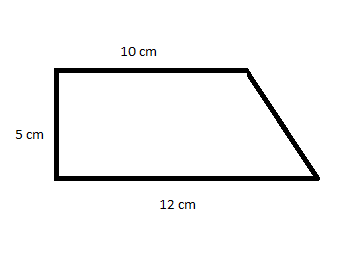55 cm2
Find the area of the shaded region: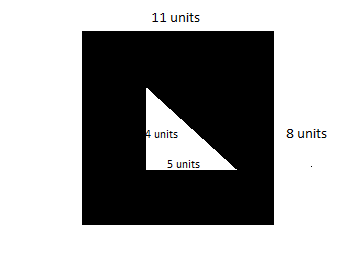78 units2
Three-Dimensional Shape
A shape that has height, width, and depth.
Prism
A three-dimensional shape that has two identical ends and non-curved sides.
Surface Area
The total area of all of the sides of a figure.
Give the surface area formula for a prism in terms of P, h, and A, where P and A represent the perimeter and area of the base respectively and h represents the height.
The formula is: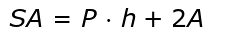Give the surface area formula for a right circular cylinder in terms of r and h, where r represents the radius and h represents the height.
The formula is: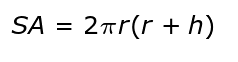If P and A represent the perimeter and area of the base respectively and s represents slant height, give the formula for surface area of a right pyramid.
The formula is: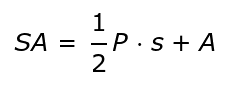Give the surface area formula for a right circular cone in terms of l and r, if l represents slant height and r represents the radius.
The formula is: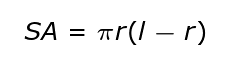Give the surface area formula for a sphere in terms of r, where r represents radius.
The formula is: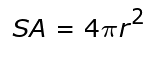An artist is painting the outside of a rectangular prism. Find the surface area if the square base has a side length of 4 inches and length of the rectangular side is 10 inches.
192 in2
Give the surface area formula of a cuboid in terms of w, h, and l, if w represents the width, h represents the height, and l represents the length.
The formula is: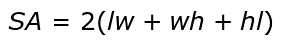Give the surface area formula for a cube in terms of a, if a represents the length of a side.
The formula is: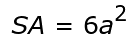Find the surface area of the figure: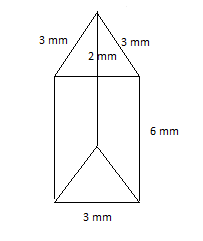60 mm2
Two-Dimensional Shape
A shape with only two measures, such as length and width, but no depth.
Volume
The amount of space taken up by an object.
Give the volume of a cube formula in terms of s, where s represents a side length.
The formula is: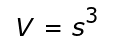Give the volume of a rectangular prism formula in terms of l, w, and h, given that l represents length, w represents width, and h represents height.
The formula is: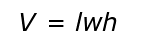Give the volume of a cylinder formula in terms of r and h, given that r represents radius and h represents height.
The formula is: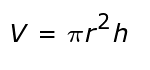Give the volume of a sphere formula for a sphere in terms of r, given that r represents radius.
The formula is: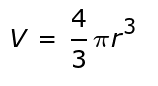Give the volume of a pyramid formula in terms of A and h, given that A represents the area of the base and h represents the height.
The formula is: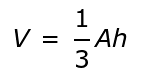Give the volume of a cone formula in terms of r and h, given that r represents radius and h represents height.
The formula is: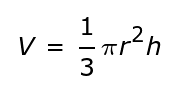Determine how many cubic inches of ice cream would fit into a cone with a radius of 3 inches and a height of 5 inches. Use 3.14 for pi.
47.1 in3
A fish tank is in the shape of a rectangular prism and its dimensions are 2 ft. by 1 ft. by 1 ft. Determine how much water the tank can hold if you are going to fill it up an inch from the top.
3,168 in3
Rectangular Prism
A three-dimensional shape where every side is a rectangle and every corner has a 90 degree angle.
Cube
A three-dimensional shape where every side is a square and all corners are 90 degrees.
Cylinder
A three-dimensional shape where the two bases are circles and the entire length has the same roundness. As an example, think of a soda can.
Sphere
A three-dimensional circular shape where every point on the surface is the same distance from the center.
Identify the shape: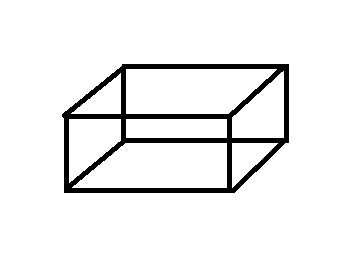Rectangular Prism
Identify the shape: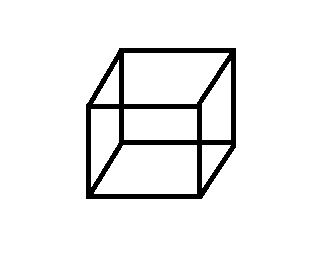Cube
Identify the shape: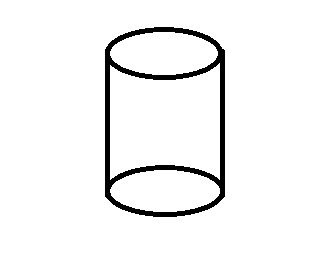Cylinder
Identify the shape: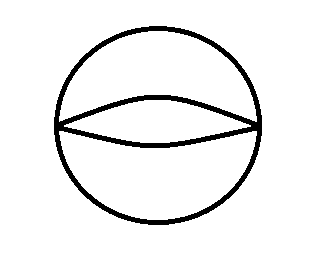Sphere
Identify the shape: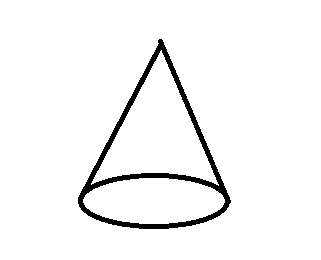Cone
Cone
A three-dimensional shape with a flat, circular base and one curved side that meets at a point.
Pyramid
A three-dimensional shape with a flat, polygon base and slanted sides that meet at a point.
Identify the shape: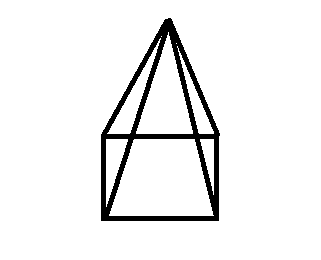Pyramid
Polyhedron
A three-dimensional shape with straight edges and flat sides.
Faces on a Polyhedron
The flat sides on the shape.
Edges on a Polyhedron
Where the edges meet on the shape.
Vertices on a Polyhedron
The corners on the shape.
Euler's Formula
The formula states that the number of faces plus the number of vertices minus the number of edges should equal 2. The formula looks like the following: F + V - E = 2.
Determine the number of faces:The figure has five faces.
Determine the number of edges:The figure has eight edges.
Determine the number of vertices:The figure has five vertices.
A particular polyhedron has four faces and six edges. Using Euler's formula, determine how many vertices it has.
The polyhedron has four vertices.
A figure with four sides.
Kite
Rhombus
A quadrilateral with four congruent sides.
Congruent
In geometry, when two things are equal.
Square
A rectangle with four congruent sides. You can also define it as a rhombus with four 90 degree angles.
Trapezoid
A quadrilateral with at least one pair of parallel lines.
Isosceles Trapezoid
A trapezoid where the non-parallel sides are congruent.
Right Trapezoid
A trapezoid where one interior angle is 90 degrees.
Parallelogram
A trapezoid with two pairs of parallel, congruent sides.
Rectangle
A parallelogram with four 90 degree angles.
Pentagon
A figure with five sides.
Hexagon
A figure with six sides.
Octagon
An eight sided figure.
Heptagon
A seven sided figure.
Regular polygon
A polygon where all sides are congruent.
Irregular Polygon
A polygon where all sides are not congruent.
Triangle
A three sided figure.
Polygon
A figure with many sides.
Ellipse
Circle
A special ellipse where the two radii are the same.
Scalene Triangle
A triangle where all three sides are different lengths.
Isosceles Triangle
A triangle where two sides are congruent.
Equilateral Triangle
A triangle where all three sides are congruent.
Right Triangle
A triangle with one 90 degree angle.
Obtuse Triangle
A triangle with one angle that is greater than 90 degrees.
Acute Triangle
A triangle with three angles that measure less than 90 degrees.
Give the best classification of the figure: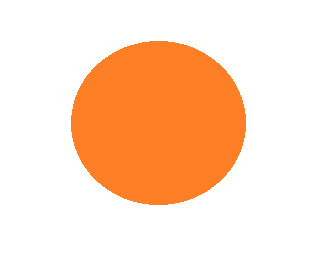Circle
Give the best classification of the figure: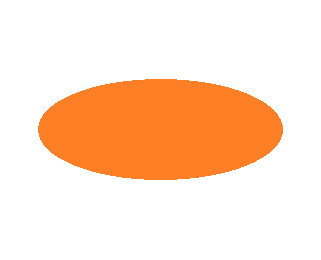Ellipse
Give the best classification of the figure: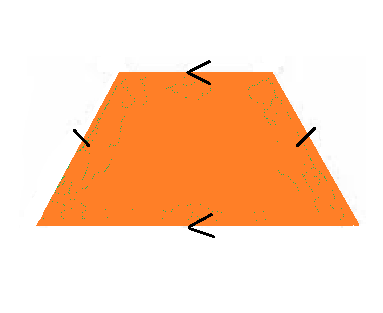Isosceles Trapezoid
Give the best classification of the figure: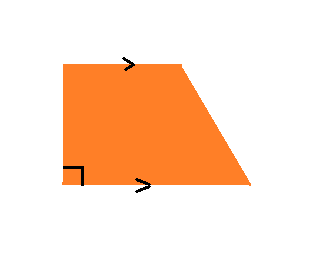Right Trapezoid
Give the best classification of the figure: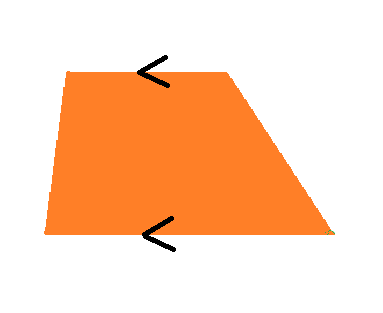Trapezoid
Give the best classification of the figure: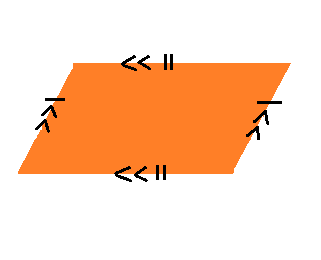Parallelogram
Give the best classification of the figure: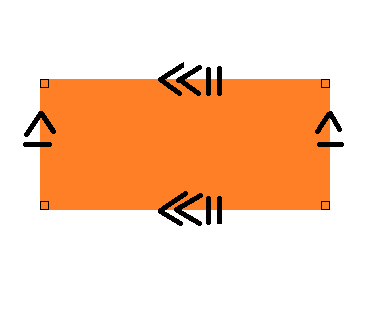Rectangle
Give the best classification of the figure: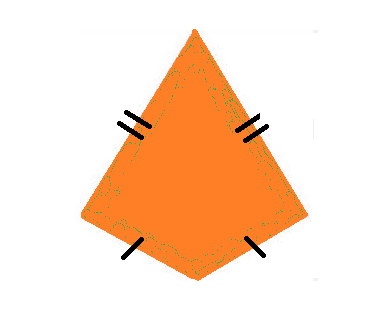Kite
Give the best classification of the figure: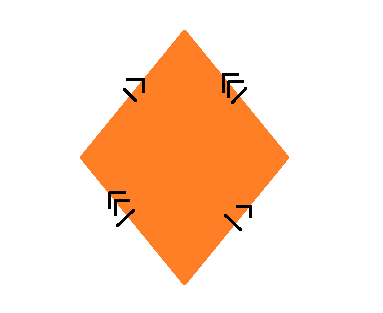Rhombus
Give the best classification of the figure: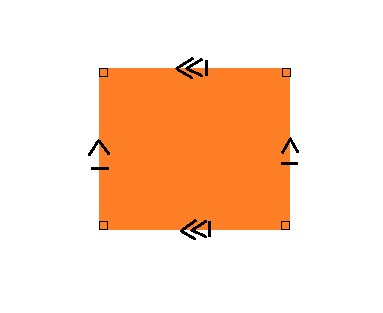Square
Identify the figure: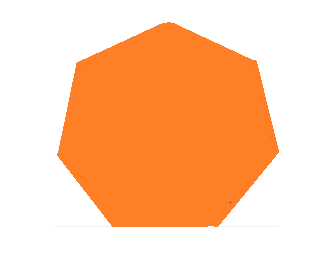Heptagon
Identify the figure:Octagon
Identify the figure:Hexagon
Identify the figure:Pentagon
Identify the triangle based on the angles: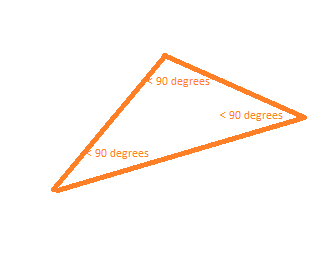Acute Triangle
Identify the triangle based on the angles: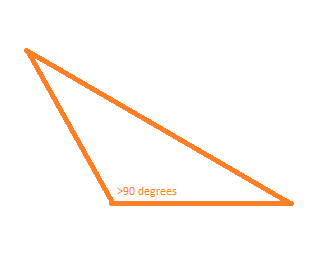Obtuse Triangle
Identify the triangle based on the angles: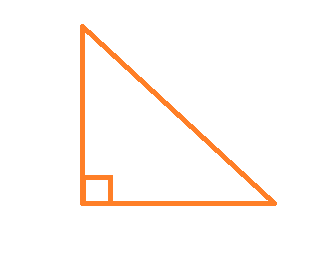Right Triangle
Identify the triangle based on the sides: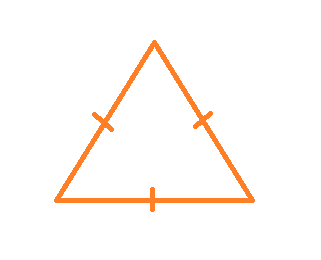Equilateral Triangle
Identify the triangle based on the sides: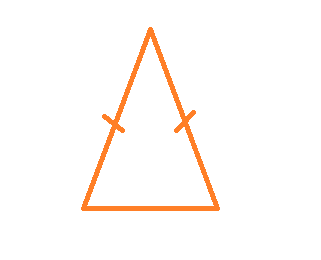Isosceles Triangle
Identify the triangle based on the sides: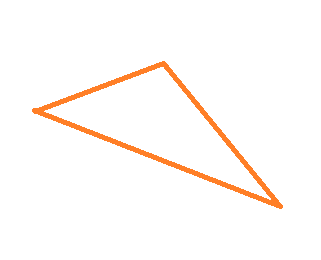Scalene Triangle

To unlock this flashcard set you must be a Study.com Member.